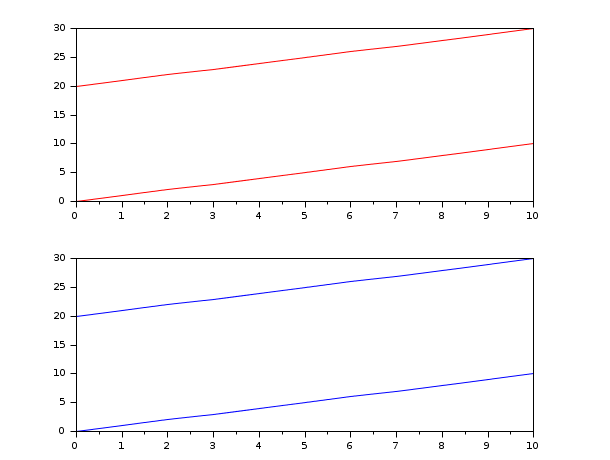Scilab Home page | Wiki | Bug tracker | Forge | Mailing list archives | ATOMS | File exchange
Change language to: English - Français - Português - Русский

See the recommended documentation of this function

Scilabヘルプ >> Statistics > Data with Missing Values > nanreglin

# nanreglin

Linear regression

### Calling Sequence

`[a,b]=nanreglin(x,y)`

### Arguments

x, y, a, b

numerical vectors or matrices.

### Description

Solve the regression problem y=a*x+b in the least square sense. `x` and `y` are two matrices of size `x(p,n)` and `y(q,n)`, where `n` is the number of samples.

The estimator `a` is a matrix of size `(q,p)` and `b` is a vector of size `(q,1)`.

Each line of `y` is treated as an independent problem, if `x` or `y` contain a NaN (`x(i,j) = %nan` or `y(i,j) = %nan`), then `x(:,j)` and `y(i,j)` are ignored, as if the point [x(:,j); y(i,j)] did not exist.

### Examples

Graphical example #1:

```// In the following example, both problems represent two straight lines:
// one goes from (0,0) to (10,10) and the other one goes from (0,20) to (10,30).
// reglin and nanreglin find the same values because all the points are aligned and the NaNs have been ignored.
subplot(211)
x = 0:10;
y = 20:30;
[a1, b1] = reglin(x, [x ; y]);
plot(x', (a1*x+repmat(b1,1,11))', "red")

subplot(212)
y2 = y;
y2(2:10) = %nan; // Leaving y2(1) and y2(11) unchanged.
[a2, b2] = nanreglin(x, [x ; y2])
plot(x', (a2*x+repmat(b2,1,11))', "blue")```Graphical example #2:

```// Now both problems represent one straight line (reglin(x, x)) from (0,0) to (2,2),
// but while the second argument of the first problem (reglin(x, y)) represents
// a flat line (with equation y = 1), the second argument of the second problem
// (reglin(x, y2)) ignores the central point of y (set to %nan) so the flat line
// now has equation y = 0, because the two remaining points are (0,0) and (2,0).
subplot(211)
x = 0:2;
y = [0 3 0];
[a1, b1] = reglin(x, [x ; y]);
plot(x', (a1*x+repmat(b1,1,3))', "red")

subplot(212)
y2 = y;
y2(2) = %nan; // y2 = [0 %nan 0];
[a2, b2] = nanreglin(x, [x ; y2]);
plot(x', (a2*x+repmat(b2,1,3))', "blue")```• reglin — 線形回帰
• pinv — 擬似逆行列
• leastsq — Solves non-linear least squares problems
• qr — QR 分解

### History

 バージョン 記述 5.5.0 Introduction